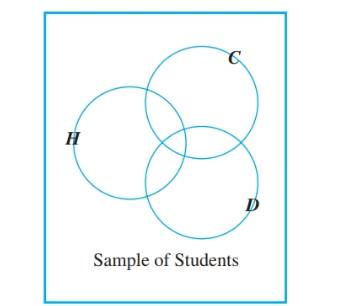Chapter 9.3, Problem 33ES### Discrete Mathematics With Applicat...

5th Edition
EPP + 1 other
ISBN: 9781337694193

#### Solutions

Chapter
Section### Discrete Mathematics With Applicat...

5th Edition
EPP + 1 other
ISBN: 9781337694193
Textbook Problem
6 views

# A college conducted a survey to explore the academic interests and achievements of its students. It asked students to place checks beside the numbers of all the statements that were true of them. Statement #1 was “I was on the Dean’s list last term,” statement #2 was I belong to an academic club, such as the math club or the Spanish club.” and statement #3 was b1 am majoring in at least two subjects.” Out of a sample of 100 students, 28 checked #1, 26 checked #2, and 14 checked #3, 8 checked both #1 and #2, 4 checked both #1 and #3, 3 checked both #2 and #3, and 2 checked all three statements. a. How many students checked at least one of the statements? b. How many students checked none of the statements? c. Let H be the set of students who checked #1, C the set of students who checked #2, and D the set of students who checked #3. Fill in the numbers for all eight regions of the diagram.d. How many students checked #1 and #2 but not #3? e. How many students checked #2 and #3 but not #1? f. How many students checked #2 but neither of the other two?

To determine

(a)

The number of students who checked at least one of the statements.

Explanation

Given information:

In a survey to evaluate the academic interest and the achievements of college students, three statements were asked from sample of 100 students.

The statements were as follows.

Statement #1 “I was on the Dean’s list last term.”

Statement #2 “I belong to an academic club.”

Statement #1 “I am majoring in at least two subjects.”

The students in the sample have responded as follows.

28 students checked the statement #1.

26 students checked the statement #2.

14 students checked the statement #3.

8 students checked both statements #1 & #2.

4 students checked both statements #1 & #3.

3 students checked both statements #2 & #3.

2 students checked all three statements.

Formula used:

The inclusion/exclusion rule for three sets.

Let A,B and C be three finite sets.

Then,

N(ABC)=N(A)+N(B)+N(C)N(AB)N(AC)N(BC)+N(ABC)

Calculation:

Let A,B and C be three finite setssuch that,

A is the student who checked the statement #1

B is the student who checked the statement #2

C is the student who checked the statement #3

Then we can summarize the given data using the set notation

To determine

(b)

The count of the students who have not checked any of the statements.

To determine

(c)

To construct a Venn diagram for the given scenario considering three sets H,C and D for the three statements.

To determine

(d)

The number of students who have checked #1 and #2 but not #3.

To determine

(e)

The number of students who checked only #2 and #3, but not #1.

To determine

(f)

The number of students who checked only #2, but not #1 and #3.

### Still sussing out bartleby?

Check out a sample textbook solution.

See a sample solution

#### The Solution to Your Study Problems

Bartleby provides explanations to thousands of textbook problems written by our experts, many with advanced degrees!

Get Started